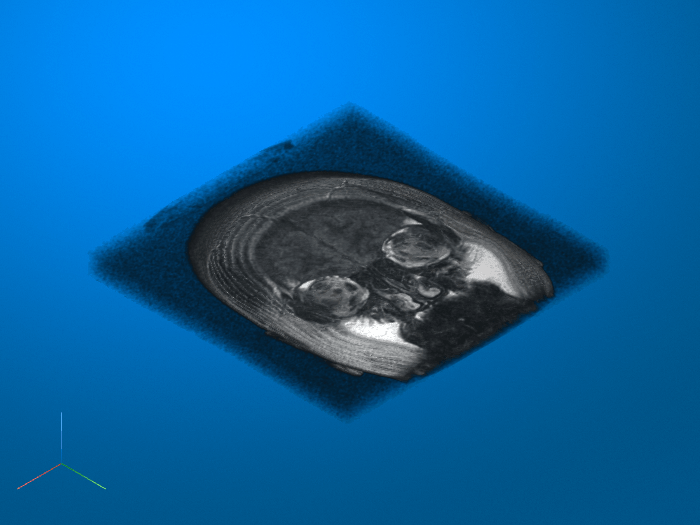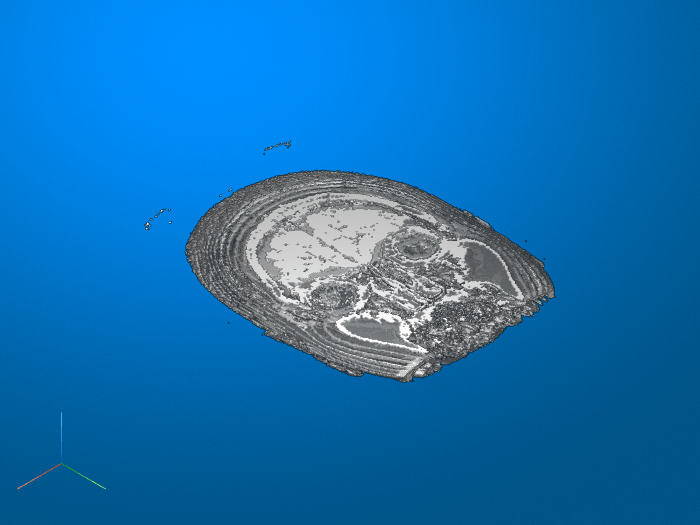imsegkmeans3

K-means clustering based volume segmentation

Description

example

L = imsegkmeans3(V,k) segments volume V into k clusters by performing k-means clustering and returns the segmented labeled output in L.

[L,centers] = imsegkmeans3(V,k) also returns the cluster centroid locations, centers.

L = imsegkmeans3(V,k,Name,Value) uses name-value pairs to control aspects of the k-means clustering algorithm.

Examples

collapse all

Load a 3-D grayscale MRI volume and display it using volshow.

volshow(mristack);Segment the volume into three clusters.

L = imsegkmeans3(mristack,3);

Display the segmented volume using volshow. To explore slices of the segmented volume, use the Volume Viewer app.

figure
volshow(L);Input Arguments

collapse all

Volume to segment, specified as a 3-D grayscale volume of size m-by-n-by-p or a 3-D multispectral volume of size m-by-n-by-p-by-c, where p is the number of planes and c is number of channels.

Note

imsegkmeans3 treats 2-D color images like 3-D volumes of size m-by-n-by-3. If you want 2-D behavior, then use the imsegkmeans function.

Data Types: single | int8 | int16 | uint8 | uint16

Number of clusters to create, specified as a positive integer.

Name-Value Arguments

Specify optional comma-separated pairs of Name,Value arguments. Name is the argument name and Value is the corresponding value. Name must appear inside quotes. You can specify several name and value pair arguments in any order as Name1,Value1,...,NameN,ValueN.

Example: 'NumAttempts',5

Normalize input data to zero mean and unit variance, specified as the comma-separated pair consisting of 'NormalizeInput' and a numeric or logical 1 (true) or 0 (false). If you specify true, then imsegkmeans3 normalizes each channel of the input individually.

Number of times to repeat the clustering process using new initial cluster centroid positions, specified as the comma-separated pair consisting of 'NumAttempts' and a positive integer.

Maximum number of iterations, specified as the comma-separated pair consisting of 'MaxIterations' and a positive integer.

Accuracy threshold, specified as the comma-separated pair consisting of 'Threshold' and a positive number. The algorithm stops when each of the cluster centers move less than the threshold value in consecutive iterations.

Output Arguments

collapse all

Label matrix, specified as a matrix of positive integers. Pixels with label 1 belong to the first cluster, label 2 belong to the second cluster, and so on for each of the k clusters. L has the same first three dimensions as volume V. The class of L depends on number of clusters.

Class of LNumber of Clusters
'uint8'k <= 255
'uint16'256 <= k <= 65535
'uint32'65536 <= k <= 2^32-1
'double'2^32 <= k

Cluster centroid locations, returned as a numeric matrix of size k-by-c, where k is the number of clusters and c is the number of channels. centers is the same class as the image I.

Tips

• The function yields reproducible results. The output will not vary in multiple runs given the same input arguments.

 Arthur, D. and S. Vassilvitskii. "k-means++: The Advantages of Careful Seeding." SODA '07: Proceedings of the Eighteenth Annual ACM-SIAM Symposium on Discrete Algorithms. New Orleans, LA, January 2007, pp. 1027–1035.